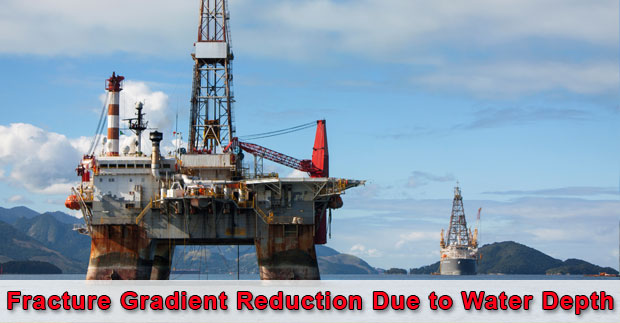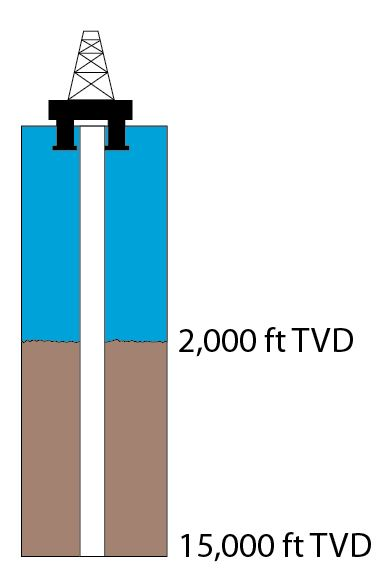# Fracture Gradient Reduction Due to Water Depth

Fracture gradient is one of the critical information which drilling engineers need to know in order to design drilling programs. For the well control stand point, the fracture gradient directly affects on how much influx volume can be successfully contained in the wellbore. If the wellbore pressure is over the fracture pressure, formations would be broken down and this situation will result in loss of drilling fluid into formations. Additionally, it might lead to well control situation because of loss of hydrostatic pressure. Fracture gradient is quite straight forward for land operation because it will not be reduce due to water column. However, the fracture gradient will be reduced in deepwater environment. In this article, we will discuss why water depth can cause the reduction in fracture gradient.Basically, the fracture gradient is related to fluids occupying in pore spaces of rock and weight of rock which are called overburden pressure. Generally, the overburden of a typical sedimentary is about 1.0 psi/ft (19.2 ppg). Rocks will be fractured when the wellbore pressure exceeds the confining stresses acting on it. If we make a general assumption that the overburden pressure causes the minimum confining stress of the rock. Then the formation fracture gradient will not be 1.0 psi/ft if the location is offshore.

Note: this assumption is made in order to help you get more understanding on how and why water depth can decrease the formation fracture gradient.

## Why does the water depth reduce fracture gradient?

Water has less density than rock and when it is calculated into overburden pressure, it will reduce overall overburden pressure. For the calculations in this article, we will use 1.0 psi/ft as the overburden of the rock.

Let’s take a look at the examples below;

1st Example – Comparison between land and offshore location at 4,000’ TVD.

Land operation at 4,000’ TVD (Figure 1)Figure 1 – Land operation at 4,000’ TVD

Overburden at 4,000’ TVD = 4,000 x 1 = 4,000 psi

Convert 4,000 psi at 4,000’ TVD in to ppg = 4,000 ÷ (0.052 x 4,000) = 19.23 ppg

Offshore operation at 4,000’ TVD with a water depth of 2,000 ft (Figure 2)

Water density is 0.45 psi/ft.Figure 2 – Offshore operation – 2000 ft water depth

Overburden pressure = (0.45 x 2,000) + (1.0 x 2,000) = 2,900 psi

Convert 2,900 psi at 4000’ TVD in to ppg = 2,900 ÷ (0.052 x 4,000) = 13.94 ppg

From the first example, you will see that at 4,000’ TVD, water depth will reduce the overburden from 19.23 ppg to 13.94 ppg.

2nd  Example – Comparison between land and offshore location at 15,000’ TVD.

Land operation at 15,000’ TVD (Figure 3)

Overburden at 15,000’ TVD = 15,000 x 1 = 15,000 psi

Convert 15,000 psi at 15,000’ TVD in to ppg = 15,000 ÷ (0.052 x 15,000) = 19.23 ppgFigure 3 – Land operation at 15,000’ TVD

Offshore operation at 15,000’ TVD with a water depth of 2,000 ft (Figure 4)

We will use the same water depth of 2,000 ft but the well depth is at 15,000’ TVD for offshore operation.Figure 4 – Offshore operation at 15,000’ TVD

Overburden pressure = (0.45 x 2,000) + (1.0 x 13,000) = 13,900 psi

Convert 2,900 psi at 4000’ TVD in to ppg = 13,900 ÷ (0.052 x 15,000) = 17.82 ppg

As you can see in the second example, the overburden of the formation still decreases due to water depth. However, it has less effect than the shallow well.

### Conclusion

Water depth will reduce the formation fracture pressure and offshore wells will have smaller margin between mud weight and fracture pressure than land wells because of water depth effect. At the same water depth, the fracture pressure at the shallower section will be decreased more than the deeper depth. What’s more, particularly at a shallow depth where the average overburden is greatly reduced by water column, more casing strings are required to reach the plan casing depth.

Share the joy1.Pedrito Ribaia says: